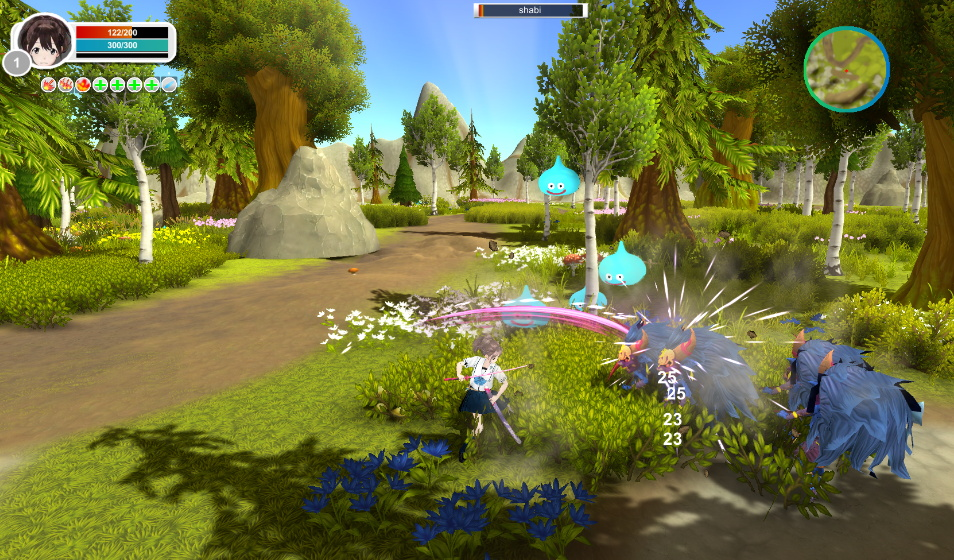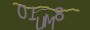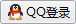# unity游戏引擎开发的ARPG游戏DEMO

demo功能包括：

8个技能（采用RT+ABXY和 LT+ABXY进行组合)

npc对话

npc任务个人比较偏爱手柄玩游戏，所以游戏按键大部分都是适配了xbox游戏手柄！增加了震动反馈，当受到打击时释放技能时手柄均会震动！使游戏玩起来更有手感！为了游戏npc更智能我使用了NPCPopulator插件制作群体npc动画，npc会随机走动在指定范围内！```IEnumerator animspeed1()
{

animator.speed = 0.1f;
yield return new WaitForSeconds(0.3f);
animator.speed = 1f;

}```

```public void OnGetEnemy(Transform player, int radius, int angle)
{

List<GameObject> mList = new List<GameObject>();
//球形射线检测,得到主角半径2米范围内所有的物件
//判断检测到的物件中有没有Enemy
if (cols.Length > 0)
{
for (int i = 0; i < cols.Length; i++)
//判断是否是怪物
if (cols[i].tag.Equals("Enemy"))
{ //判断敌人是否在主角前方60度范围内

Vector3 dir = cols[i].transform.position - player.position;
if (Vector3.Angle(dir, player.forward) < angle)
{

}
}

float distance_min = 10000;    //主角与怪物的最近距离
float distance = 0;            //当前怪物与主角的距离
int id = 0;                    //与主角最近的怪物的编号
//遍历所有敌人,计算距离并比较
for (int i = 0; i < mList.Count; i++)
{

distance = Vector3.Distance(transform.position, mList[i].transform.position);
if (distance < distance_min)
{
//找到一个更近的
distance_min = distance;
id = i;

}
}

if (mList.Count > 0)
{

Vector3 targetPos = mList[id].transform.position;
//攻击点面向怪物

targetPos.y = this.transform.position.y;
this.transform.LookAt(targetPos);//使主角望向距离最近的敌人并开始攻击
targetPos.y = targetPos.y + 1;
attackPoint.LookAt(targetPos);

}

// cols[i].GetComponent<BaseHero>().OnTakeDamage();

}
return;
}```

``` IEnumerator SetVibration(float right, float left, float tim)
{
if (this.tag == "Player") {
Debug.Log("震动");
// XInputDotNetPure.dll
yield return new WaitForSeconds(tim);
}```### 精致女战士模型免费分享

POLYGONS: 49772 论坛搜集的一个精美的女战士3dmax模型，免费分享给大家拿去做练习！模型是国外大神制作的，全部采用的2048尺寸的高清贴图，并且烘焙的低模不会过多消耗pc资源...

### 浮光_燕尔枯荣模型带绑定下载

《天涯明月刀》是腾讯游戏自主研发的首款3D武侠题材的MMORPG游戏，采用自主研发的Quicksliver引擎，是比肩国际顶尖水平的次世代网络游戏。其场景描绘了浪漫与现实之间广袤天地，造型...

### 精美古埃及风格女孩人物模型 Egypt Girl角色模型

#### 发表评论◎欢迎参与讨论，请在这里发表您的看法和观点。